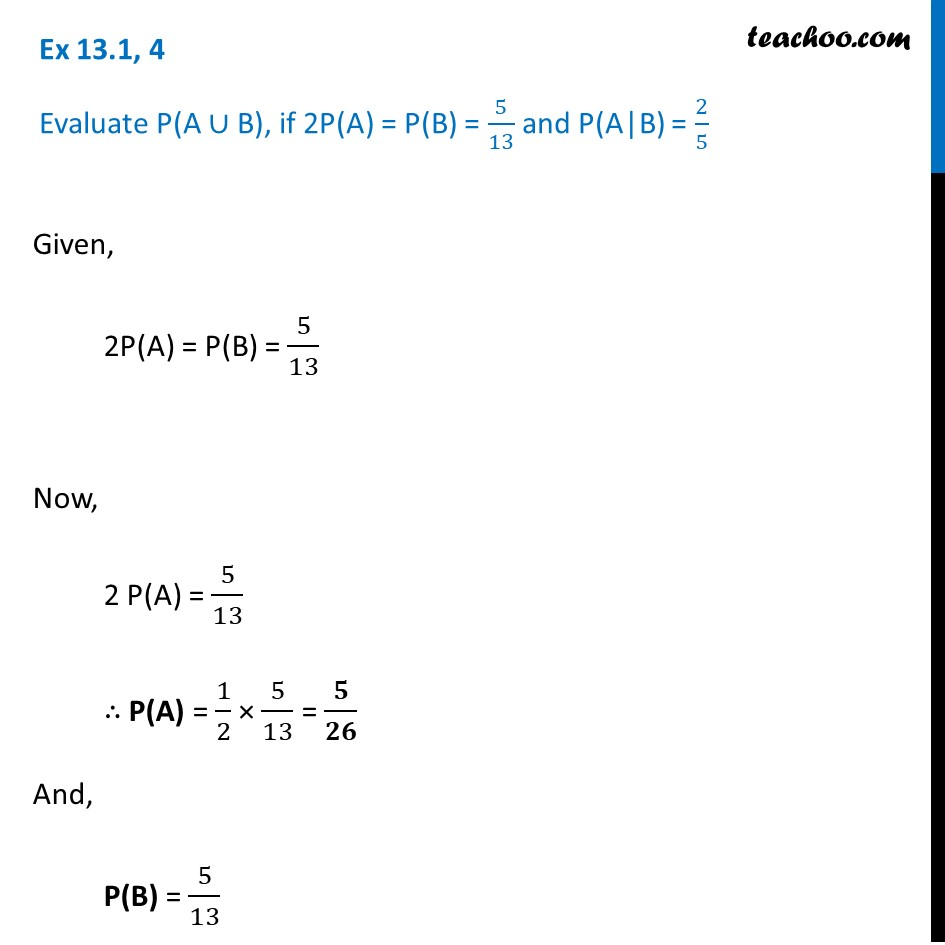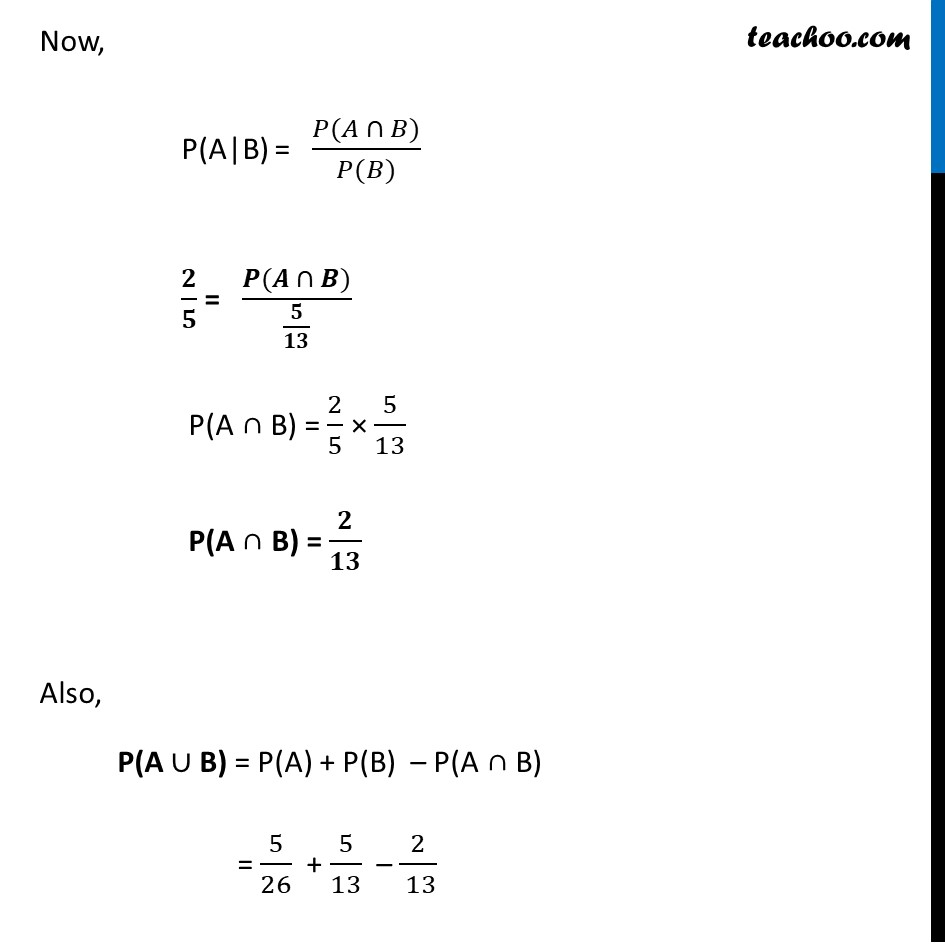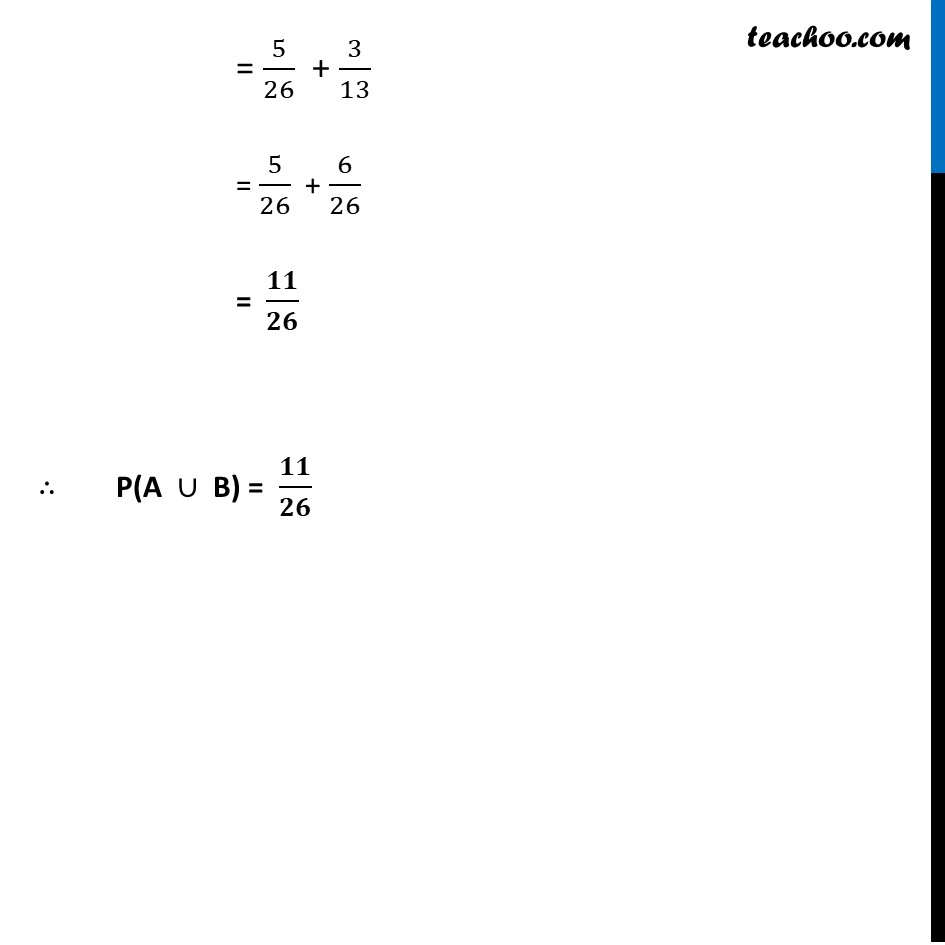Ex 13.1

Chapter 13 Class 12 Probability
Serial order wiseLearn in your speed, with individual attention - Teachoo Maths 1-on-1 Class

### Transcript

Ex 13.1, 4 Evaluate P(A ∪ B), if 2P(A) = P(B) = 5/13 and P(A|B) = 2/5Given, 2P(A) = P(B) = 5/13 Now, 2 P(A) = 5/13 ∴ P(A) = 1/2 × 5/13 = 𝟓/𝟐𝟔 And, P(B) = 5/13 Now, P(A|B) = (𝑃(𝐴 ∩ 𝐵))/(𝑃(𝐵)) 𝟐/𝟓 = (𝑷(𝑨 ∩ 𝑩))/(𝟓/𝟏𝟑) "P(A ∩ B)" = 2/5 × 5/13 "P(A ∩ B)" = 𝟐/𝟏𝟑 Also, "P(A"∪"B)" = P(A) + P(B) – "P(A ∩ B)" = 5/26 + 5/13 – 2/( 13) = 5/26 + 3/13 = 5/26 + 6/26 = 𝟏𝟏/𝟐𝟔 ∴ "P(A "∪" B)" = 𝟏𝟏/𝟐𝟔